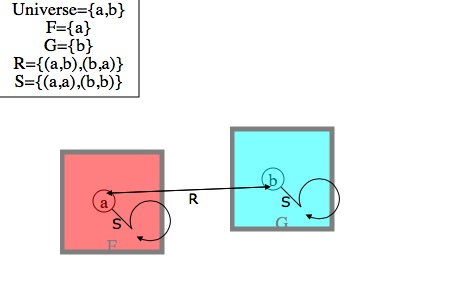# Tutorial 23: The semantics of relations

Logical System

### The Tutorial

The semantics of relations proceeds in much the way one would expect-- the new item that has to be taken account of is the order of the terms (because, for example, Tab is not at all the same thing as Tba -- Arthur being taller than Beryl is not the same as Beryl being taller than Arthur).

Interpretation 1

Universe= {a,b}
F={a}

Remember, there needs to be an account of how the various symbols in the predicate logic formulas apply in this Universe. There are the constant terms a,b,c... -- at an introductory level we can just let a name a, b name b etc. There are the predicates -- here we just have to say which members of the Universe a predicate applies to, and this can be done by writing, for example, F={a} to mean that a has the property F; many properties will not apply to any of the objects in the Universe, if, for example, G applies to nothing, this could be written G={} or nothing need be written at all.

There are also now the relations -- here we just have to say which members of the Universe a relations applies to, and this can be done by writing, for example, R={<ab>} to mean that a bears the relation R to b (in that order); many relations will not apply to any of the objects in the Universe, if, for example, S applies to nothing, this could be written S={} or nothing need be written at all.

[There are two alternative common notations for ordered pair: <ab> is one, and (a,b) is the other. We prefer <ab>, however angle brackets forming a tag, say <h1>, is common html, xml, svg, etc. and these are all technologies used extensively here. So, to avoid some issues with programming we occasionally use (a,b).]

Now consider

Interpretation 2

Universe= {a,b}
F={a}
R={<ab>}

The formula Rab is true under Interpretation 2. The formula Rba is false under Interpretation 2. The formula Sab is false under Interpretation 2. The formula Rab∧Fa is true under Interpretation 2. The formula (∃x)Rax is true under Interpretation 2. And so on...

## Exercise to accompany Predicate Tutorial 13.

The Interpretation in Predex13 shows an Interpretation similar to thisif it does not, draw a similar one yourself. The diagrammatic conventions are as follows. The named circles are individuals; the rectangles show properties, which individuals may or may not have; relations are shown as a labeled arrow from one individual to another; it is possible for a relation to related an individual to itself (as in Jim likes himself i.e. Ljj), to depict this the labeled drawn arrow follows part of a circle back on itself. The Intepretation Board (top-left) shows exactly what the interpretation is.]

Exercise 1(of 1)

Interpretations Applet